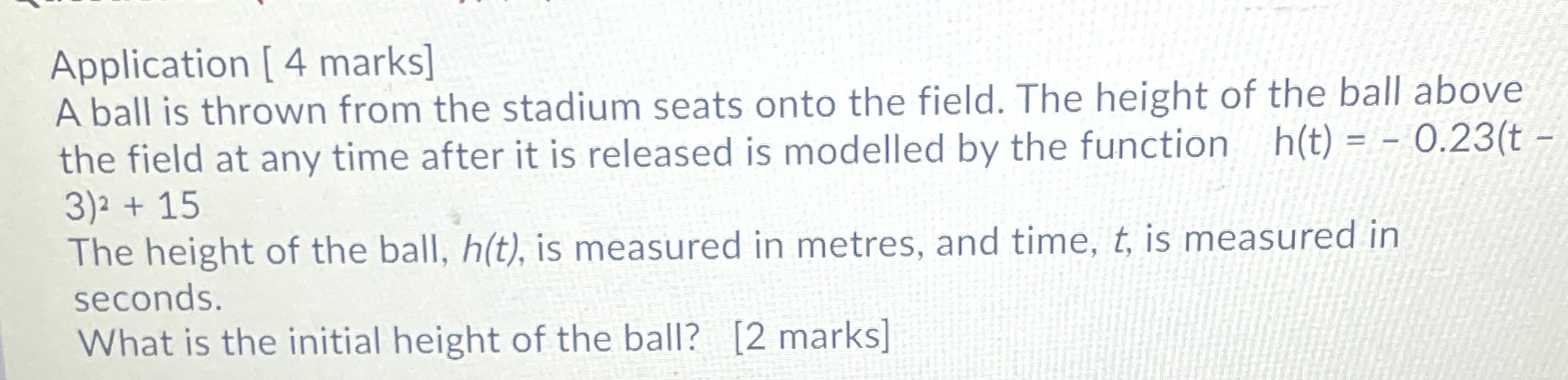### ¿Todavía tienes preguntas de matemáticas?

Pregunte a nuestros tutores expertos
Algebra
PreguntaA ball is thrown from the stadium seats onto the field. The height of the ball above the field at any time after it is released is modelled by the function $$h ( t ) = - 0.23 ( t -$$ $$3 ) ^ { 2 } + 15$$

The height of the ball, $$h ( t )$$ , is measured in metres, and time, $$t$$ , is measured in seconds.

What is the initial height of the ball?